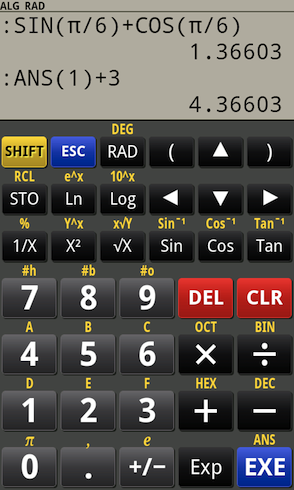# PG Calculator for Android

## PG Calculator is a powerful scientific and financial calculator for Android with customizable user interface.

PG Calculator is an excellent replacement for a standard calculator.

PG Calculator works in Algebraic and RPN modes.

PG Calculator is available in three different versions: Free, Standard and Pro.

PG Calculator allows to use mathematical functions described below:

• Addition, subtraction, multiplication and division;
• Power and roots;
• Trigonometric functions: sin, cos, tan, asin, acos, atan;
• Hyperbolic functions;
• natural and decimal logarithms;
• factorials;
• conversion between binary, octal, hexadecimal, decimal and scientific (exponential) number formats;
• Time Value of Money solver;
• IP Subnet Calculator;

There is a library of common mathematical and physical constants which can be used in calculations in both RPN and Algebraic modes. PG Calculator also allows to use user-defined variables in expressions.

PG Calculator has built-in Time Value of Money solver and IP Subnet Calculator.

The user interface of the calculator can be changed by using various skins supplied with the application.

PG Calculator is available for following platforms:

• Android 1.6 or higher;

You may also consider looking at PG Calculator for Windows and LinuxTweet Passionate about Candles Android AppsCopyright (C) 2011-2014 P.Gridniew